Ex 12.1

Chapter 12 Class 7 Algebraic Expressions
Serial order wise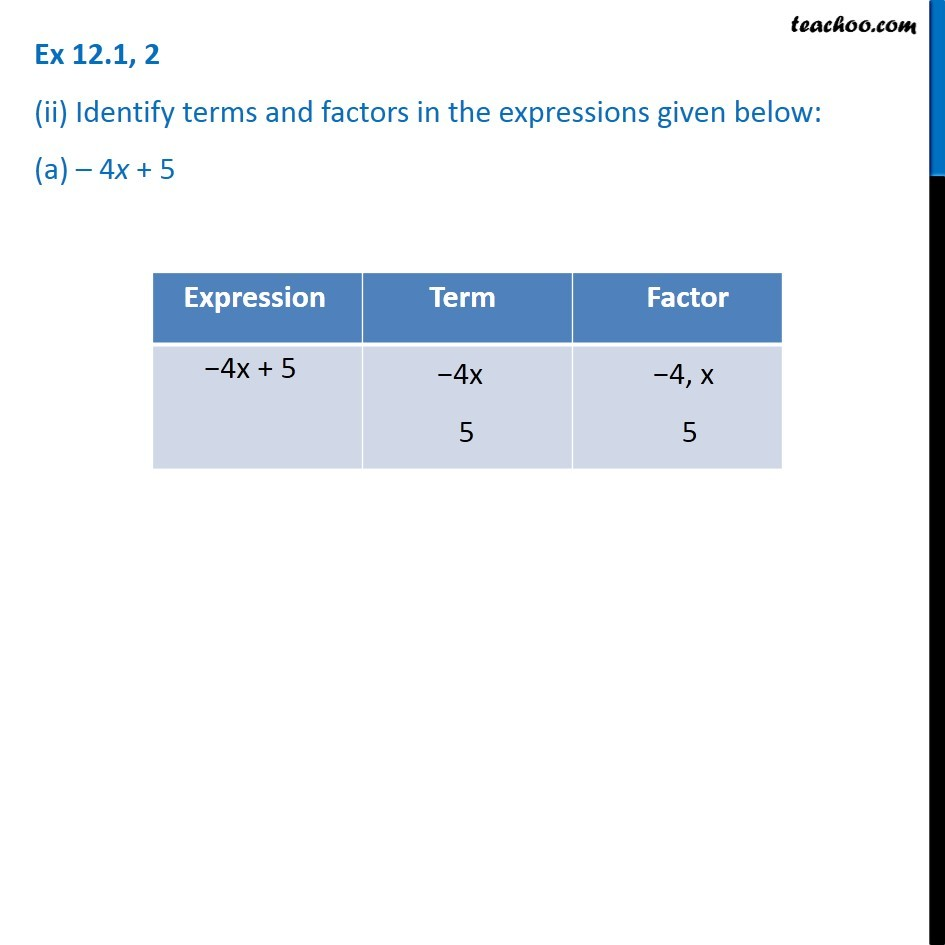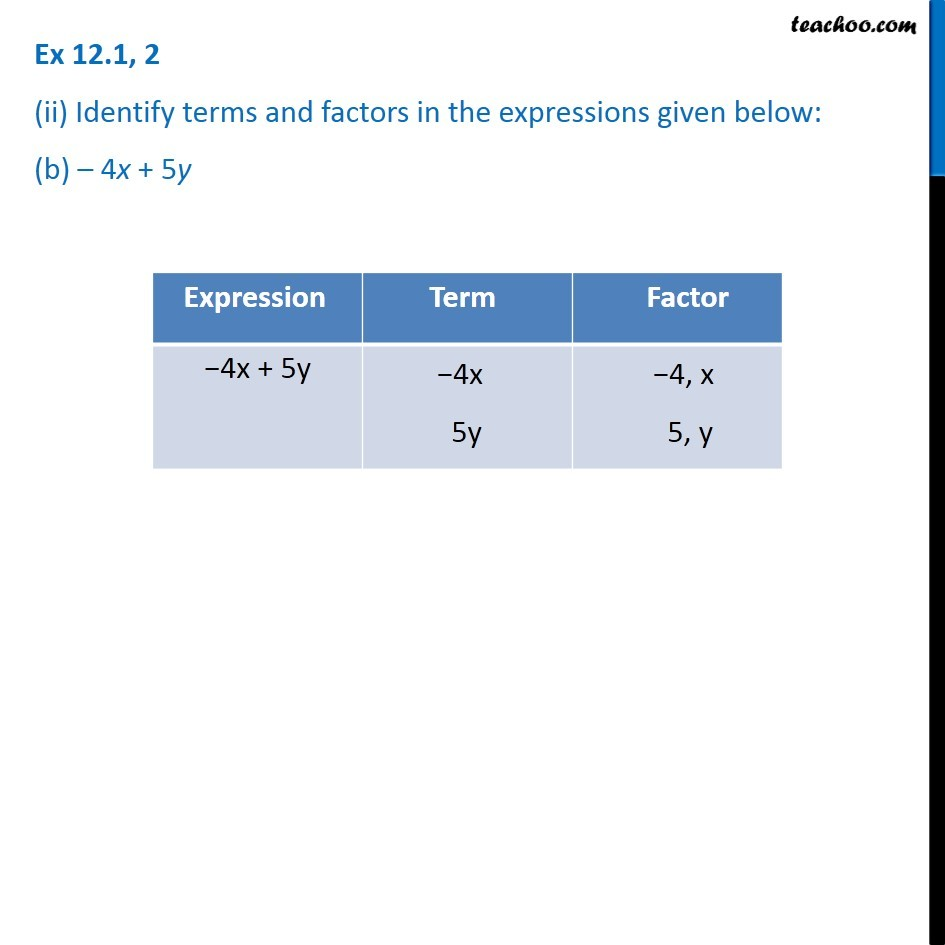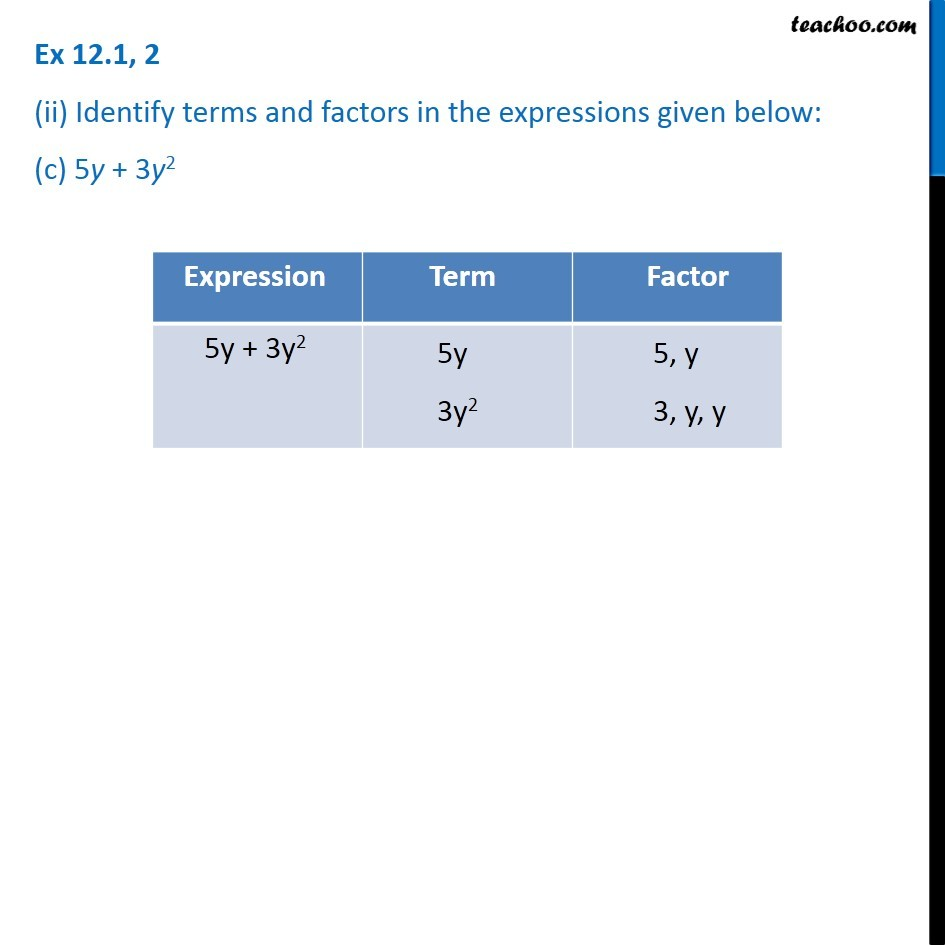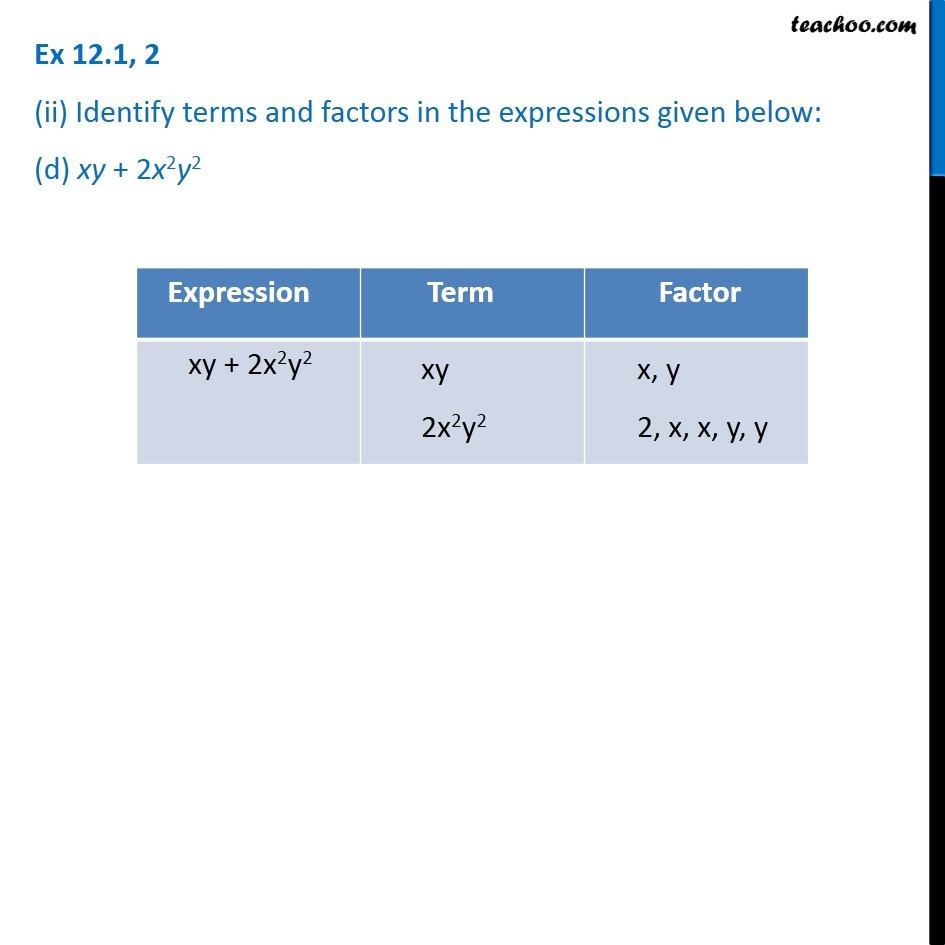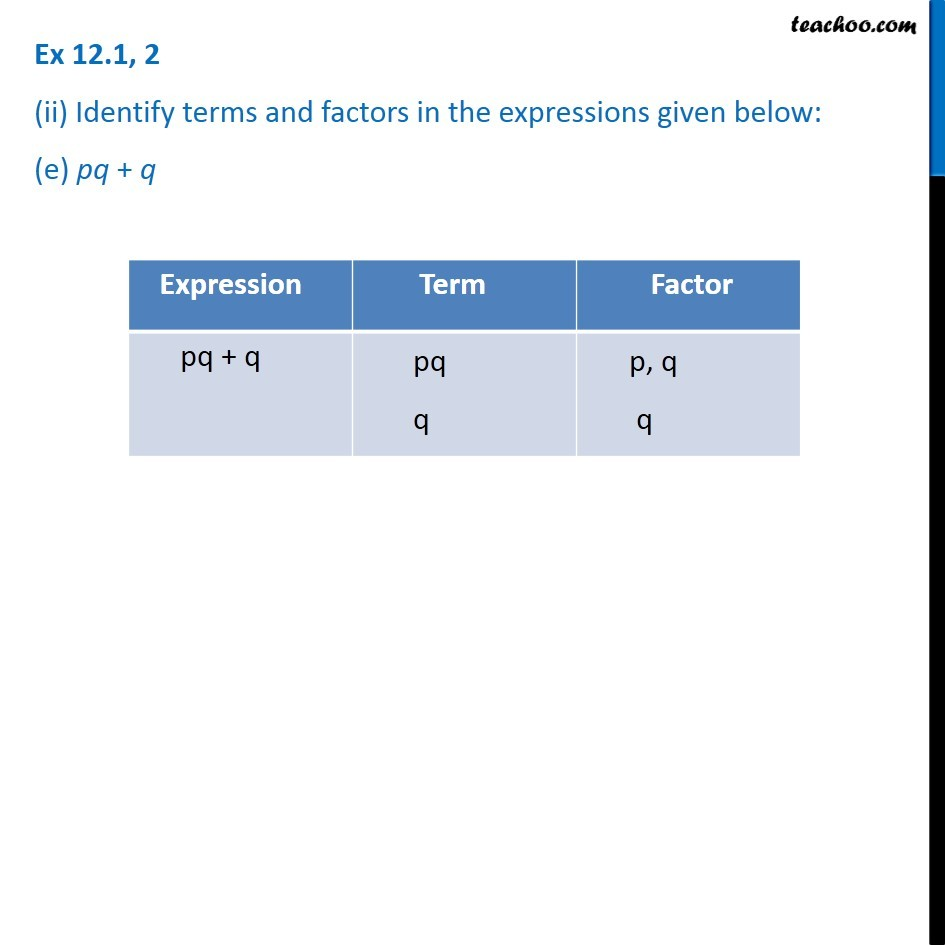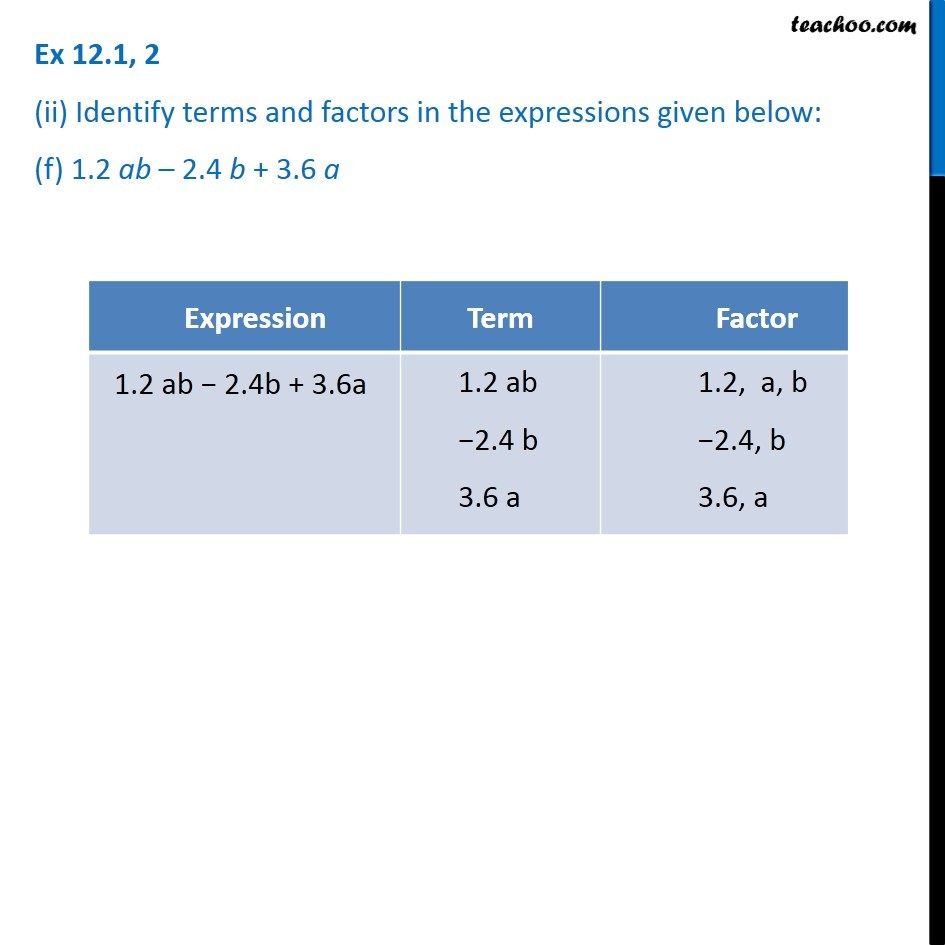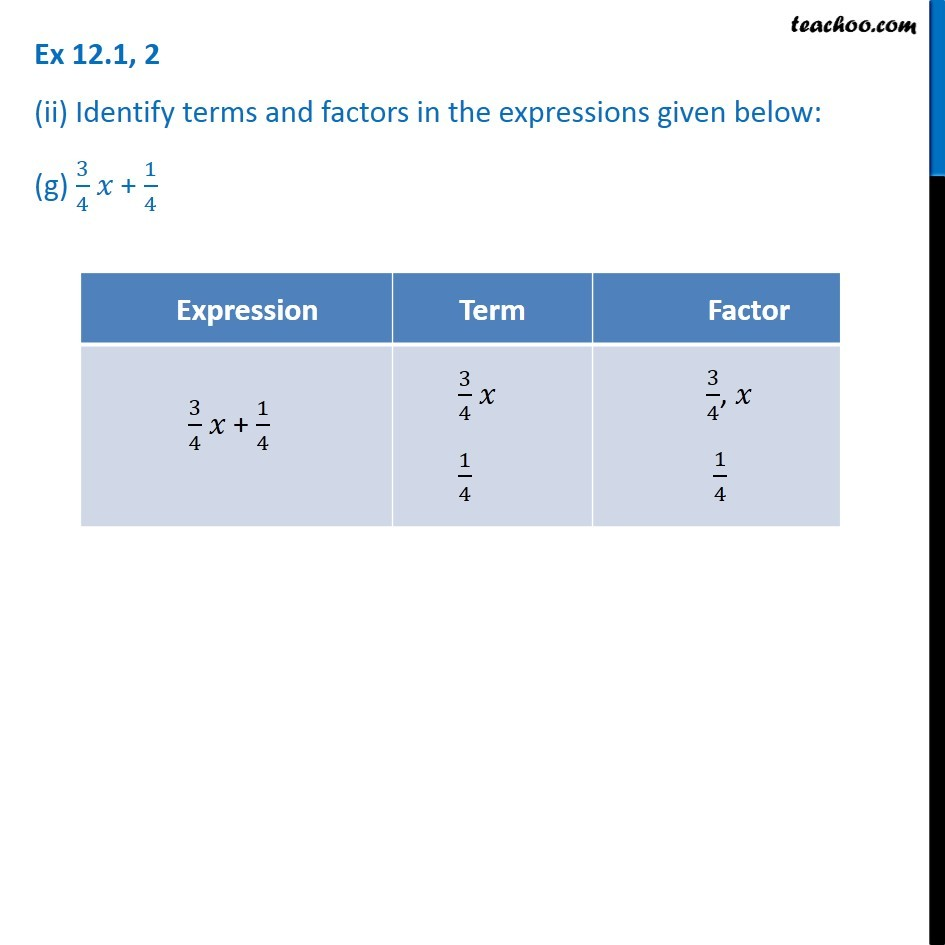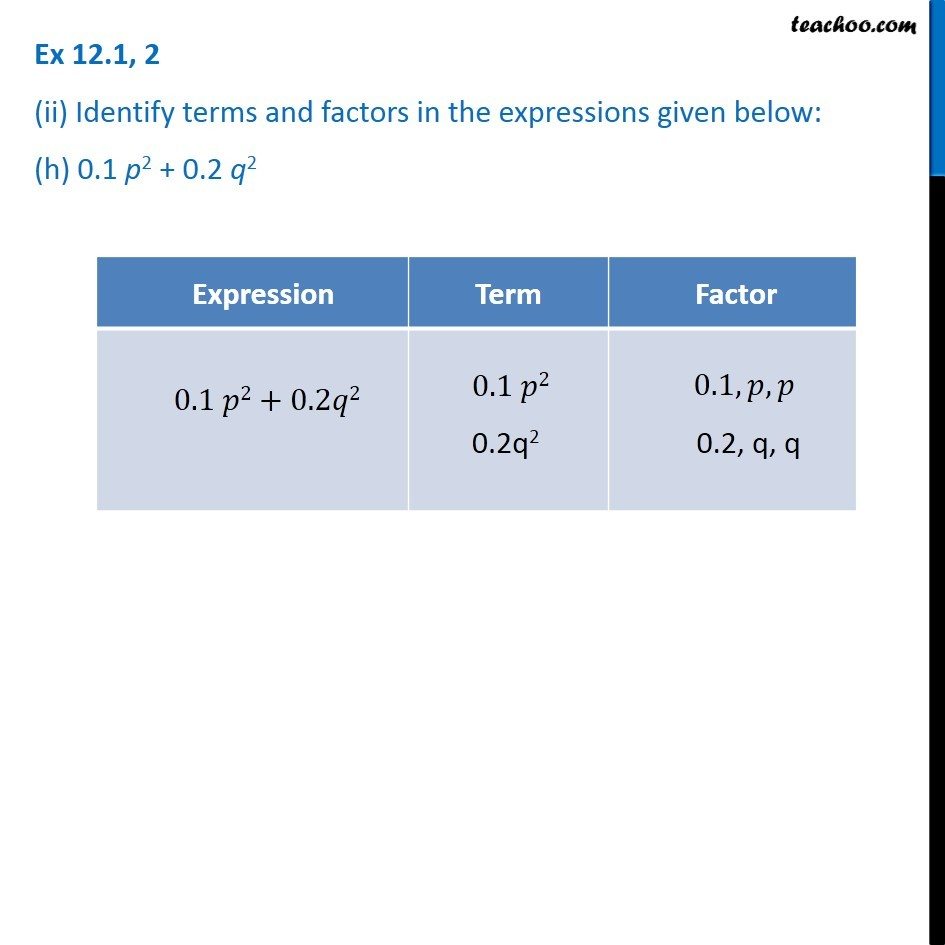Introducing your new favourite teacher - Teachoo Black, at only ₹83 per month

### Transcript

Ex 12.1, 2 (ii) Identify terms and factors in the expressions given below: (a) – 4x + 5 Ex 12.1, 2 (ii) Identify terms and factors in the expressions given below: (b) – 4x + 5y Ex 12.1, 2 (ii) Identify terms and factors in the expressions given below: (b) – 4x + 5y Ex 12.1, 2 (ii) Identify terms and factors in the expressions given below: (c) 5y + 3y2 Expression Term Factor -4x + 5 4x -4x 3 5 Ex 12.1, 2 (ii) Identify terms and factors in the expressions given below: (b) – 4x + 5y Ex 12.1, 2 (ii) Identify terms and factors in the expressions given below: (c) 5y + 3y2 Ex 12.1, 2 (ii) Identify terms and factors in the expressions given below: (e) pq + q Ex 12.1, 2 (ii) Identify terms and factors in the expressions given below: (f) 1.2 ab – 2.4 b + 3.6 a Ex 12.1, 2 (ii) Identify terms and factors in the expressions given below: (g) 3/4 𝑥 + 1/4